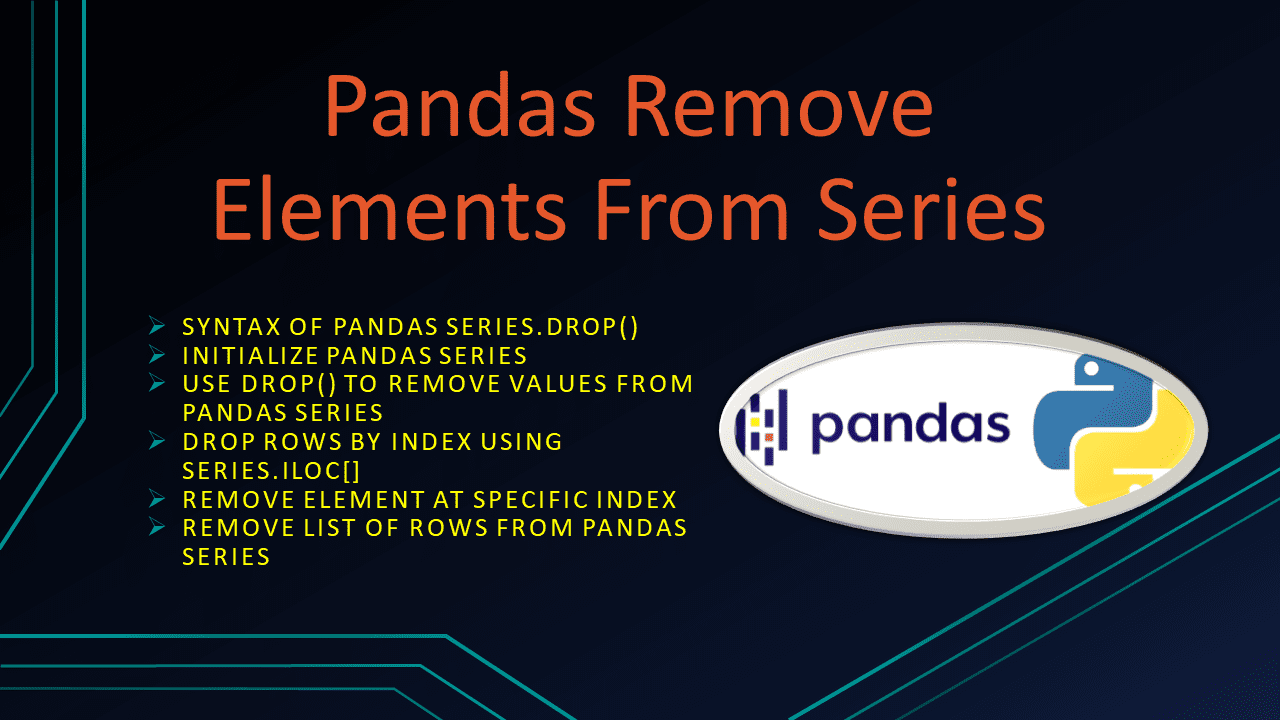# Pandas Remove Elements From Series

• Post author:
• Post category:Pandas

Pandas `Series.drop()` function is used to drop/remove elements from Series in Python. It returns the Series with the specified index labels removed. In this article, I will explain how to remove elements from the Series in Pandas by using `drop()` and other methods with examples.

## 1. Quick Examples of Remove Elements From Series

If you are in hurry below are some quick examples of the remove elements from a series in python.

``````
# Below are the quick examples

# Example 1: Use Series.drop() function
# to remove values from series
ser2 = ser.drop(labels = ['Java','Spark','NumPy'])

# Example 2: Drop rows by index use series.iloc[]
ser2 = ser.iloc[2:]

# Example 3: Use drop() function to remove rows
ser2 = ser.drop(ser.index)

# Example 4: Remove list of rows from pandas series
ser2 = ser.drop(ser.index[[1, 3, 5]])

# Example 5: Using slice interval
ser2 = ser.drop(ser.index[2:5])
``````

## 2. Syntax of Pandas Series.drop()

Following is the syntax of `Series.drop()` function.

``````
# Syntax of Series.drop()
Series.drop(labels=None, axis=0, index=None, columns=None, level=None, inplace=False, errors='raise')
``````

### 2.1 Parameters of drop()

Following are the parameters of drop() to remove the elements from pandas Series.

• `lables` – single label or list-like, Index labels to drop.
• `axis` – {0 or ‘index’} : Redundant for application on Series.
• `index` – Redundant for application on Series, but the index can be used instead of labels.
• `columns` – Use ‘index’ or ‘labels’ instead.
• `level` – int or level name, optional, For MultiIndex, the level for which the labels will be dropped.
• `inplace` – bool, default False: If True, do operation inplace and return None.
• `errors` – {‘ignore’, ‘raise’} Default Value: ‘raise’, If ‘ignore’, suppress the error and only existing labels are removed.

### 2.2 Return value of drop()

The drop() returns a Series with specified index labels removed.

## 3. Initialize Pandas Series

Pandas Series is a one-dimensional, Index-labeled data structure available only in the Pandas library. It can store all the datatypes such as strings, integers, float, and other python objects. We can access each element in the Series with the help of corresponding default indices.

Now, let’s create pandas series using list of values.

``````
import pandas as pd

# Create the Series
ser = pd.Series([20000,25000,23000,28000,55000,23000,28000])

# Create the Index
index = ['Java','Spark','PySpark','Pandas','NumPy','Python',"Oracle"]

# Set the index
ser.index = index
print(ser)
``````

Yields below output.

``````
# Output:
Java       20000
Spark      25000
PySpark    23000
Pandas     28000
NumPy      55000
Python     23000
Oracle     28000
dtype: int64
``````

## 4. Use drop() to Remove Values from Pandas Series

You can use the Pandas `Series.drop()` function to drop the single element by Index or multiple elements by index from the list.

``````
# Use Series.drop() function to remove values from series
ser2 = ser.drop(labels = ['Java','Spark','NumPy'])
print(ser2)
``````

Yields below output.

``````
# Output:
PySpark    23000
Pandas     28000
Python     23000
Oracle     28000
dtype: int64
``````

## 5. Drop Rows by Index using Series.iloc[]

By using `Series.iloc[]` property we can slice which index we want to remove from the Series. The below example drops the 3rd value. For example.

``````
# Drop rows by index use series.iloc[]
ser2 = ser.iloc[2:]
print(ser2)
``````

Yields below output.

``````
# Output:
PySpark    23000
Pandas     28000
NumPy      55000
Python     23000
Oracle     28000
dtype: int64
``````

## 6. Remove Element at Specific Index

By combining drop() function and index, we can also remove rows from the pandas series at the specified index. Here I am removing the fourth element from the series.

``````
# Use drop() function to remove rows
ser2 = ser.drop(ser.index)
print(ser2)
``````

Yields below output.

``````
# Output:
Java       20000
Spark      25000
PySpark    23000
NumPy      55000
Python     23000
Oracle     28000
dtype: int64
``````

## 7. Remove List of Rows From Pandas Series

Let’s also see how to remove multiple elements from the pandas Series by Index number. For instance, the list [1,3,5] are passed into drop() function which will remove the specified list of rows from the Series.

``````
# Remove list of rows from pandas series
ser2 = ser.drop(ser.index[[1, 3, 5]])
print(ser2)
``````

Yields below output.

``````
# Output:
Java       20000
PySpark    23000
NumPy      55000
Oracle     28000
dtype: int64
``````

Alternatively, using the drop() function with help of the slicing technique we can remove the rows along with the specified intervals. For examples.

``````
# Using slice interval
ser2 = ser.drop(ser.index[2:5])
print(ser2)
``````

Yields below output.

``````
# Output:
Java      20000
Spark     25000
Python    23000
Oracle    28000
dtype: int64
``````

## 8. Complete Examples of Remove Element From Pandas Series

``````
import pandas as pd

# Create the Series
ser = pd.Series([20000,25000,23000,28000,55000,23000,28000])

# Create the Index
index = ['Java','Spark','PySpark','Pandas','NumPy','Python',"Oracle"]

# Set the index
ser.index = index
print(ser)

# Use Series.drop() function to remove values from series
ser2 = ser.drop(labels = ['Java','Spark','NumPy'])
print(ser2)

# Drop rows by index use series.iloc[]
ser2 = ser.iloc[2:]
print(ser2)

# Use drop() function to remove rows
ser2 = ser.drop(ser.index)
print(ser2)

# Remove list of rows from pandas series
ser2 = ser.drop(ser.index[[1, 3, 5]])
print(ser2)

# Using slice interval
ser2 = ser.drop(ser.index[2:5])
print(ser2)
``````

## 9. Conclusion

In this article, I have explained how to remove elements from a series by using `Series.drop()`, `series.iloc[]`, and `Series.index()` functions with examples.

Happy Learning !!

### Naveen

I am a Data Engineer with 20+ years of experience in transforming data into actionable insights. Over the years, I have honed my expertise in designing, implementing, and maintaining data pipelines with frameworks like Apache Spark, PySpark, Pandas, R, Hive and Machine Learning. My journey in the field of data engineering has been a continuous learning, innovation, and a strong commitment to data integrity. I have started this SparkByExamples.com to share my experiences with the data as I come across. You can learn more about me at LinkedIn